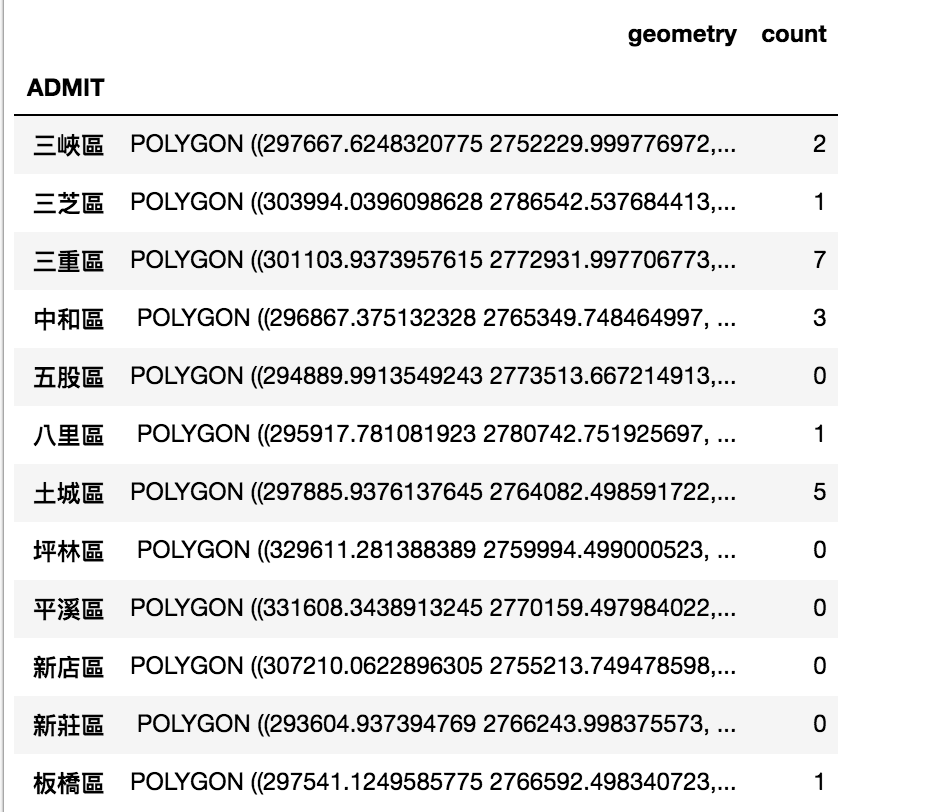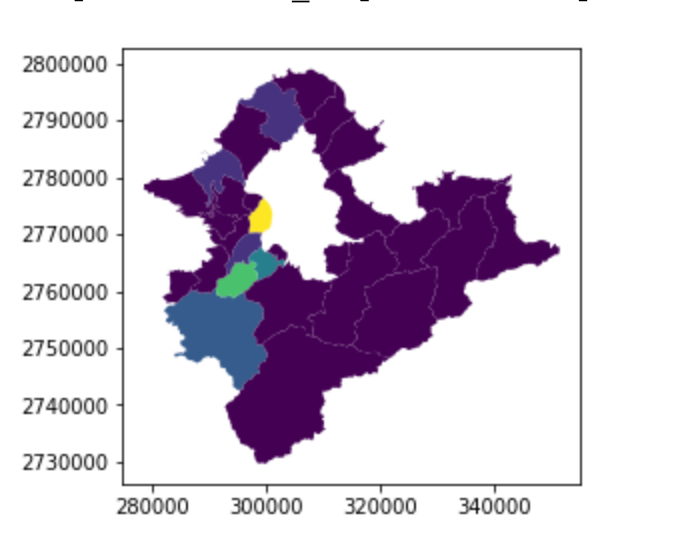#2019 iT 邦幫忙鐵人賽DAY 5
3
AI & Data

## Day05 基本的資料聚合

• groupby
• spatial join
• spatial join準備
• spatial join操作
• aggregation
• dissolve

## groupby

(其實是路燈主管機關啦，但我們先當作行政區...)

``````light=gpd.read_file('output/light.shp',encoding='utf-8')
light
``````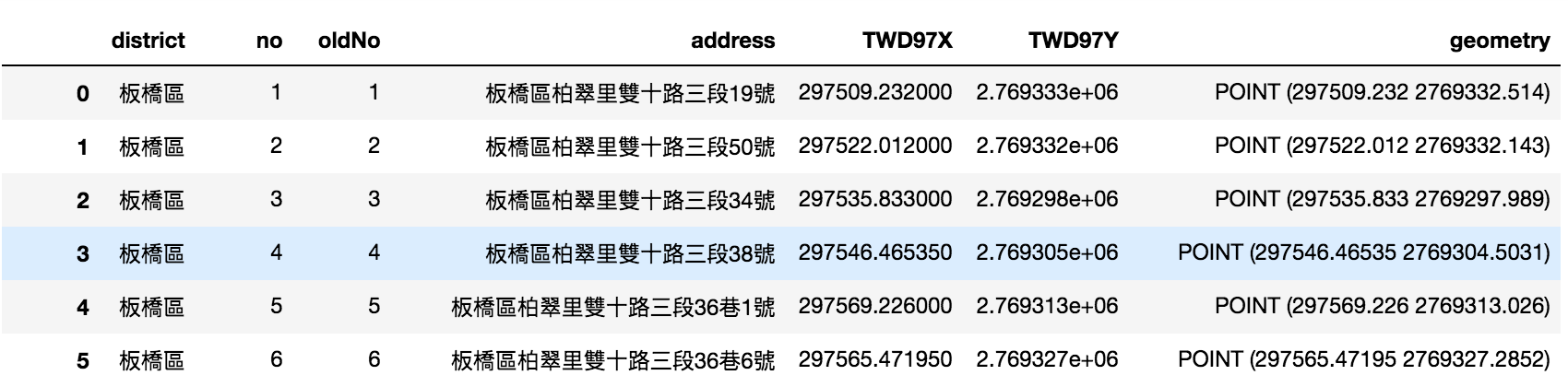GeoPandas這部分跟Pandas一樣，

``````light.groupby('district').size()
``````## spatial join

### spatial join準備

``````import geopandas as gpd
village.crs= {'init' :'epsg:3826'} # 避免資料沒設，這邊再重新給一次
``````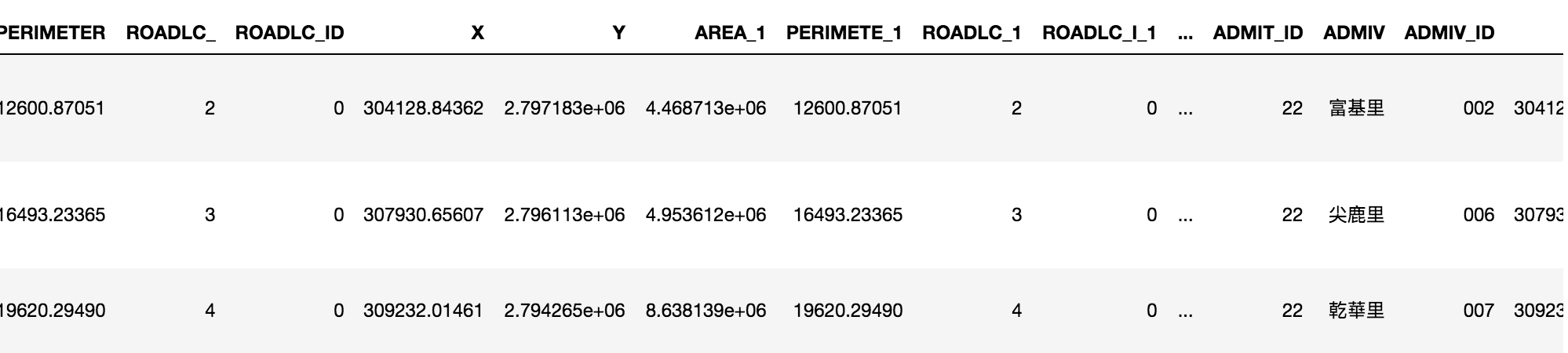``````lib=gpd.read_file('output/library.shp',encoding='utf-8')
lib.crs= {'init' :'epsg:4326'} # 避免資料沒設，這邊再重新給一次
lib=lib.to_crs(epsg=3826)
``````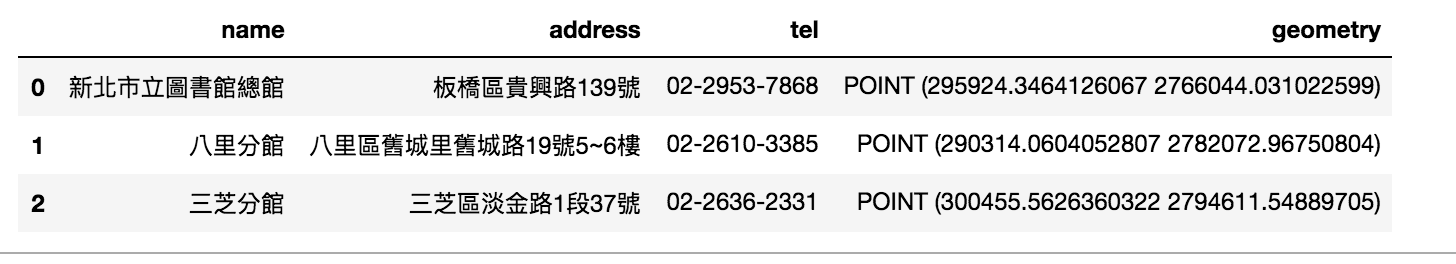### spatial join操作

(ps.為了方便顯示，只選擇`中和區`)

``````base=village[village['ADMIT']=='中和區'].plot(color='yellow')
``````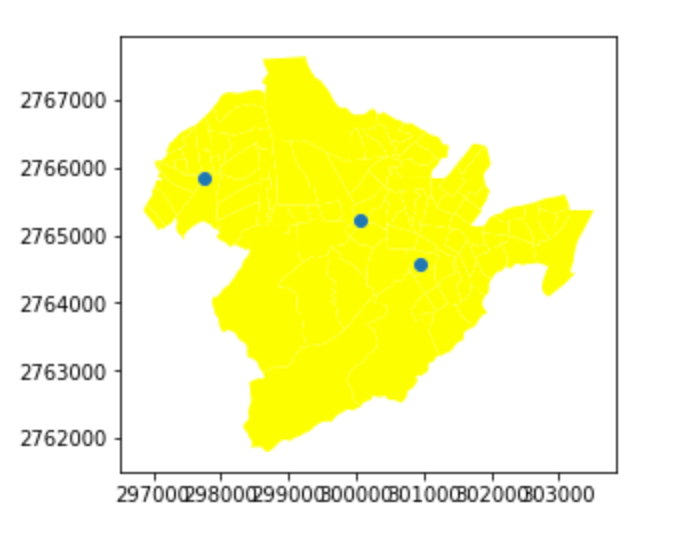``````result = gpd.tools.sjoin(lib, village[['ADMIV','ADMIT','geometry']], how="left")
result
``````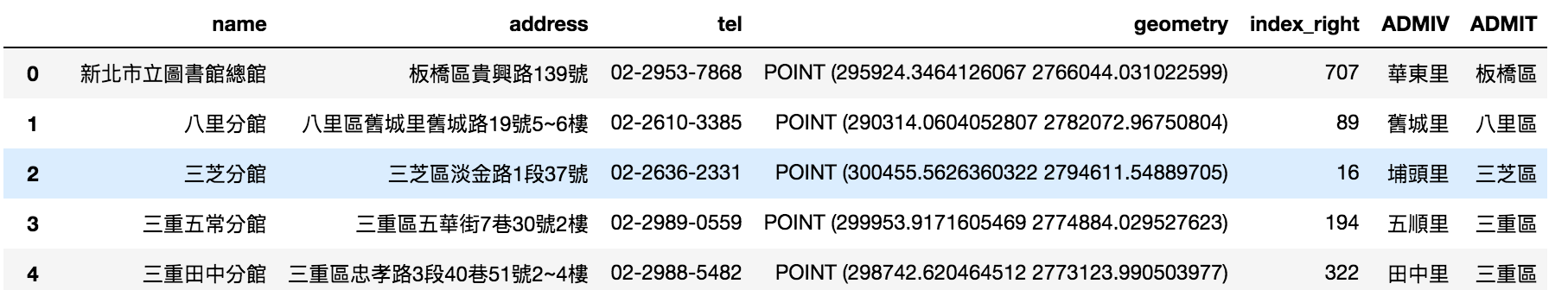spatial join完成後，我們就可以做groupy，達到前面的效果

``````group=result.groupby(['ADMIT','ADMIV'])
group.size().reset_index(name='counts')
``````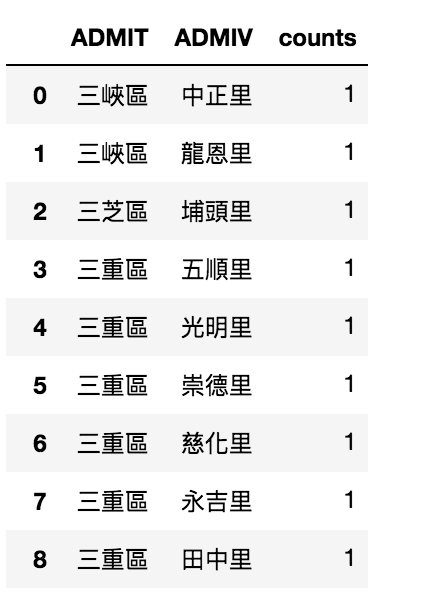``````result.plot('ADMIT')
``````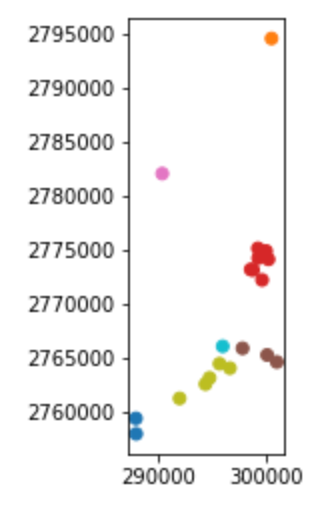## aggregation

``````import numpy as np
counts=[np.sum(row['geometry'].intersects(lib.unary_union)) for idx, row in village.iterrows()]
village['count']=counts
aggre_v[aggre_v['count']>0]
``````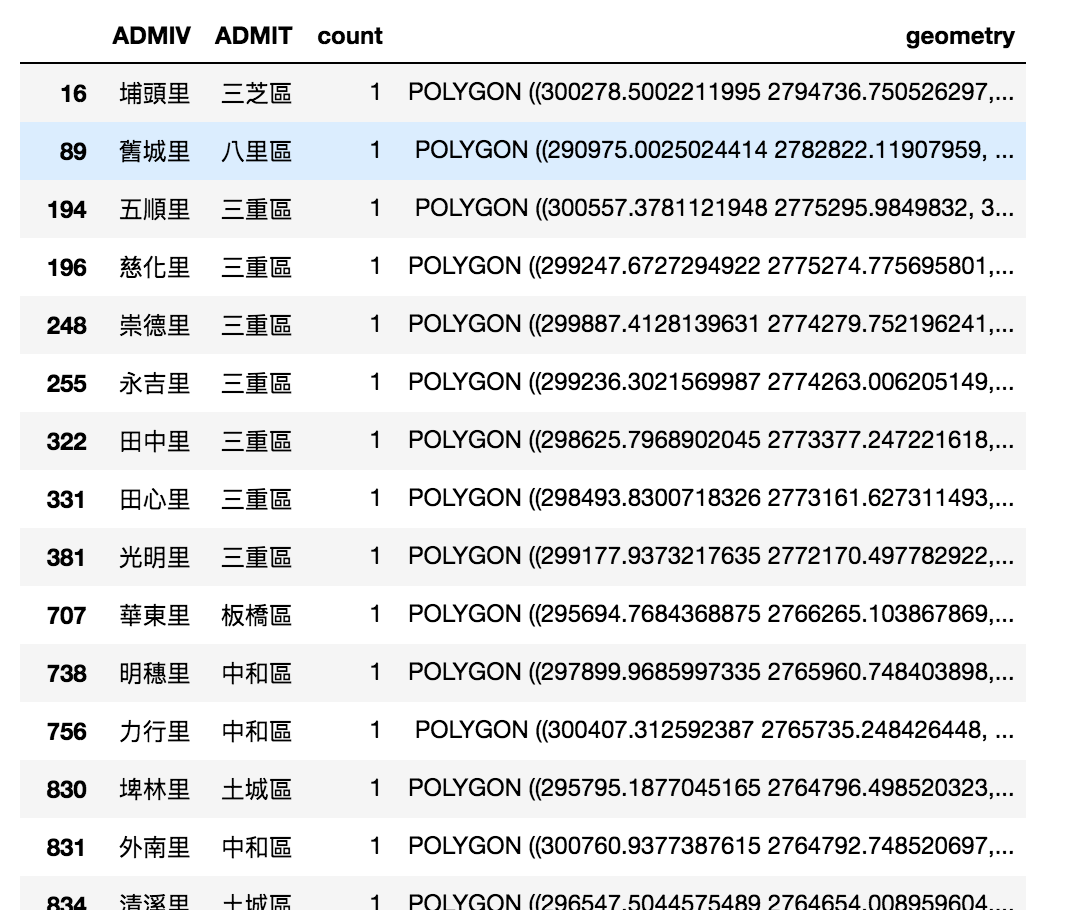``````aggre_v.plot('count')
``````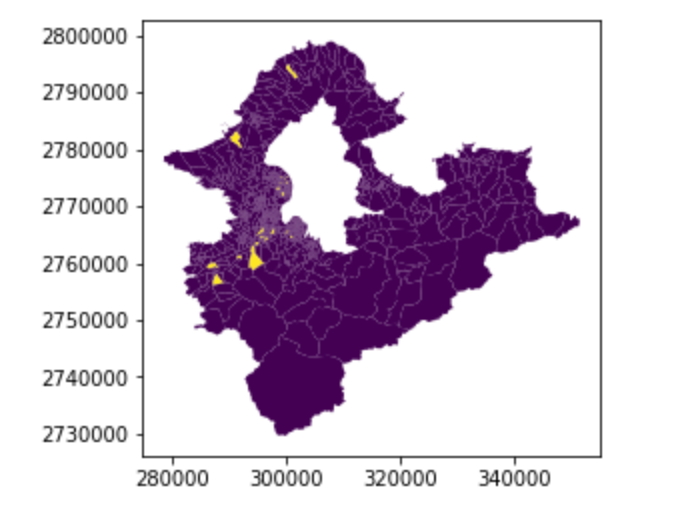## dissolve

``````dis=aggre_v.dissolve('ADMIT',aggfunc='sum')
dis
``````